Courses

# Chapter Notes, Probability, Class 9 Mathematics Class 9 Notes | EduRev

## Class 9 : Chapter Notes, Probability, Class 9 Mathematics Class 9 Notes | EduRev

The document Chapter Notes, Probability, Class 9 Mathematics Class 9 Notes | EduRev is a part of Class 9 category.
All you need of Class 9 at this link: Class 9

INTRODUCTION

In our daily life, we say that there is a probability that something will happen when it is likely to happen. We often use phrases such as :
"There is a probability that it will rain today",
"There is a probability that it will be a hot day tomorrow",
"There is a probability that he may be right", etc.
The statements:
"It will rain today."
"It will be a hot day tomorrow."
"He may be right."
are the events (or situations) about which have an element of uncertainty in our mind, that the event may happen or may not happen. The happening of an event rules out the possibility of the non-happening of the event and vice-versa. The element of uncertainty about the happening and non-happening of an event can be measured mathematically. The branch of Mathematics, which deals with it, is called Theory of Probability.

HISTORY OF PROBABILITY THEORY
In old times, dice games was a common mode of gambling. In 1654, a French gambler Chevalier De-Mere, approached the well known 17th century French philosopher and mathematician Blaise Pascal (1623-1662) regarding some dice problems. Pascal discussed this problem with another French mathematician, Pierre De-Fermat (1601-1665). Both these mathematicians solved the problem independently. This work of Pascal and Fermat laid the foundation of the probability theory.

The first book on the subject was published in 1663. The title of the book was "Book on Games of Chance".
Significant contributions in the field were also made by mathematicians J. Bernoulli (1654-1705), P. Laplace (1749- 1827), A.A. Markov (1856-1922) and A.N. Kolmogorov (in the 20th century).

DIFFERENT APPROACHES
There are following three approaches to theory of probability:
(i) Experimental approach or Empirical approach or Observed frequency approach.
(ii) Classical approach.

(iii) Axiomatic approach.
Here, in this chapter, we shall study only the Empirical probability and not the other two approaches. The remaining two kinds of approaches are to be left for the time being and we will study these in the next classes.

RANDOM EXPERIMENT
In theory of probability, we deal with situations which are outcomes of a random experiment. This experiment is of a different nature in comparison to the experiment made in physical sciences. Here, an experiment repeated under same conditions, may not give the same result (or outcome). For example, we' toss a coin and we get head but there is no guarantee that we must have the same result on the second toss. In this case, it may be tail.

When we perform a random experiment, it is called a trial. For example, if we toss a coin (fair coin) once, the possible outcomes (i,e., results) are H and T. Here, H means head and T means tail. Now, we toss a pair of two similar fair coins and the possible outcome of one toss, i.e., one trial are:

(i) Tail on both the coins, i.e., no head.

(ii) Tail on one coin and head on the other coin, i.e., exactly one head and one tail.

The above outcomes can be expressed in numerical numbers as 0, 1, 2. So, there are three possible outcomes of one trial of tossing two coins simultaneously and these outcomes are written as 0, 1, 2. Here, 0 means that no head occurs ; 1 means that exactly one head occurs ; and 2 means that exactly two heads occur. There is no possibility of any other value or outcome of this random experiment.
Similarly, if we toss three similar (fair) coins simultaneously, then the possible outcomes will be 0, 1, 2 and 3.

TOSSING OF A DIE AND ITS POSSIBLE OUTCOMES
A die is a fair and balanced cube with its six faces marked with dots from one to six as below: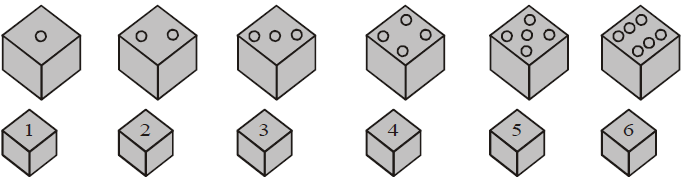If a die is tossed, we find one face out of the six faces at the top. We count the number of dots on this face.
The outcome is 'n' if the number of dots on the top face are n ; n = 1, 2, 3, 4, 5, 6. Thus, in this random
experiment, there are six possible outcomes. The possible outcomes are :
1. i.e., we get 1 on the top face ;
2. i.e., we get 2 on the top face ;
3. i.e., we get 3 on the top face ;
____________________________
____________________________
6, i.e., we get 6 on the top face.

EVENT ASSOCIATED WITH A RANDOM EXPERIMENT
An event associated with a random experiment is a collection of some outcomes out of the possible outcomes of the random experiment. Events are generally denoted by the capital letters A, B, E, F, etc.
For example, a pair of coins are tossed simultaneously and the possible outcomes are, 0 number of heads, 1 head and 2 heads. If E be the event of getting at least one head, then E = {1, 2}. Here we say that the outcomes 1 and 2 of the random experiment favours the happening of the event E but the outcome 0 does  not favour the happening of the event E or in other words, the outcome 0 favours the happening of the event 'not E'. A teacher gives four coins (fair) of same type to a student and asks him throw these four coins on the floor. The teacher makes enquiry about the number of heads from the other student. The correct answer given by that student is as below :

Either 0 number of heads (all four are tails)
or 1 head (other three are tails)
or 2 heads (other two are tails)
or 3 heads (one is tail)

Now, the teacher say that an event E is to get more than 1 heads. The third student is asked by the teacher to name the outcomes which favour the happening of the event E.
The third students reply is as under:
The favourable outcomes are 2, 3 and 4.
We can write E = {2, 3, 4}.

Two students play a game by throwing a die. Student X says that he will win if he gets an even number on
the die and he will lose the game to the second student Y if the outcome is more than 4. Now, the question
arises, who is more beneficial, X or Y ? Let us examine the truth.

The possible outcomes are 1, 2, 3, 4, 5 and 6.
Here, X wins the game if the outcomes are 2, 4 and 6, i.e., the three outcomes favours X. But if the outcomes
are of values more than 4, then X loses the game and Y wins the game. Such outcomes are 5 and 6. Thus,
only two outcomes favours Y. Clearly X is more beneficial than Y.

In the above activity, event E is to get an even number and the favourable outcomes are 2, 4 and 6. Now,
we denot the event of getting a number greater than 4 as F. So, the favourable outcomes for the, happening
of the event F are 5 and 6.

DATA OF MORE THAN ONE TRIALS
A single trial as a random experiment gives exactly one outcome at a time. On this basis, we cannot claim
that the occurence of all outcomes have equal chances. We will confirm, through practical activity, that the chances for the happening of all outcomes is equally likely.

Practical Activity
Take a fair coin, toss it 50 times and record the outcome after every toss in the form of values H (for head)
and T (for tail). Here, the number of trials are 50 and the data has two distinct values as H and T. Now, prepare
a frequency distribution table as below :
Outcomes Number of times i.e., frequency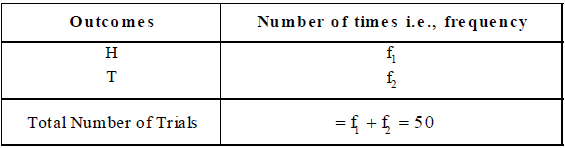Observe the values of the fractions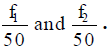. You will notice the following :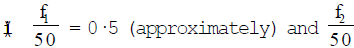i.e., the possibility of the occurences of head and tail is equally likely for each.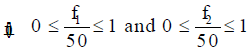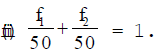Practical Activity
Toss a pair of coins simultaneously 50 times and record the outcomes as 0, 1 and 2 for each toss or trial. As
usual, 0 means no head and all tails; 1 means one head and one tail; and 2 means two heads and no tail.
Now, prepare a frequency distribution table as below: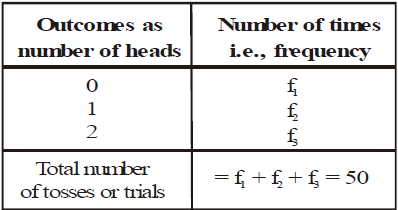Observe the values of the fractions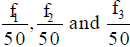. You will notice the following:

(i) All the fractions are approximately equal to (1over 3) , i.e., 0.33....., equally likely chances for the happening of
0, 1 and 2.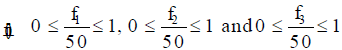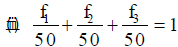EMPIRICAL PROBABILITY OF AN EVENT
In practical activity 1, the values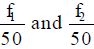are the empirical probabilities for the happening of the outcomes
H and T respectively.
In practical activity 2, the values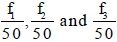are the empirical probabilities for the happening of the outcomes 0, 1 and 2 respectively. In this activity, if we say that the event E happens when we get atleast one head and then prepare frequency distribution table for two events which are E and not E as below :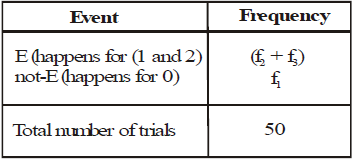Here, the values of the fractions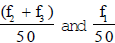are empirical probabilities of the events E and not-E respectively.
For example, if we toss a pair of coins 20 times and we get 0, 7 times ; 1, 7 times; and 2, 6 times, we
have the table for the events E and not-E as below :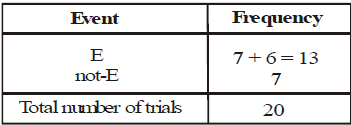Probability of the event E = (13over 20) and probability of the event not-E =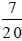Also, we find that Probability of E + Probability of not-E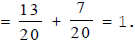P (E) denotes the empirical probability for the happening of an event E. In this chapter, we shall use word probability in place of empirical probability. We define,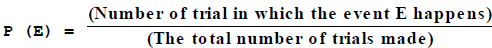REMARK 1. P (E) + P (not-E) = 1.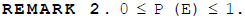REMARK 3. Sum of the probabilities of all the outcomes of a random experiment is 1.

Sure Event
When all the outcomes of a random experiment favour an event, the event is called a sure event and its empirical probability is 1.

Impossible Event
When no outcome of a random experiment favours an event, the event is called an impossible event and its
empirical probability is 0.

Ex.1 There are 40 students in a class and their results is presented as below :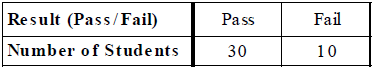If a student chosen at random out of the class, find the probability that the student has passed
the examination.
Sol.
Total number of chances = 40
Chances or trials which favour a student to pass = 30
The probability of the required event, i.e., the student has passed the examination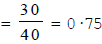Ex.2 A coin is tossed 150 times and the outcomes are recorded. The frequency distribution of the outcomes H (i.e., head) and T (i.e., tail) is given below :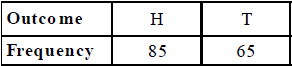Find the value of P (H), i.e., probability of getting a head in a single trial.

Sol. Total number of trials = 150
Chances or trials which favour the outcome H = 85.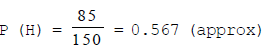Ex.3 A die is tossed 120 times and the outcomes are recorded as below: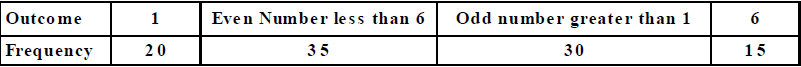Find the probability in a trial of getting
(i) The number 1

(ii) The number 6
(iii) The even number less than 6.

(iv) The odd number greater than 1.
Sol.
Total number of trials = 120
(i) Chances which favour the outcome 1 are 20.
So, P (getting 1)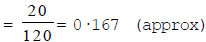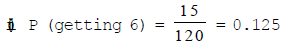(iii) Now, getting an even number less than 6 implies that the outcomes 2 or 4.
The total number of chances of getting 2 or 4, i.e., an even number less than 6 = 35
P (even number less than 6)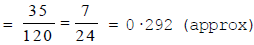(iv) Getting an odd number greater than 1, i.e., 3 or 5.
The chances favouring an odd number greater than 1 = 30.
So, P (an odd number greater than 1)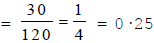Ex.4 Two similar coins were tossed simultaneously 1000 times and the frequency distribution of heads
obtained on each toss is as below :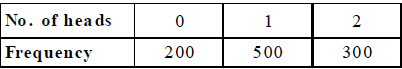Find the probabilities of the following :

(i) Probability of getting one head.

(ii) Probability of getting two heads.

(iii) Probability of getting at least one head

(iv) Probability of getting less than two heads.

(v) Probability of getting three heads.

(vi) Probability of getting not more than two heads.

Sol. Total number of trials = 1000
(i) Total number of chances favouring the event of getting one head = 500. So, P (one head)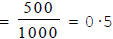(ii) P (two heads)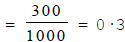(iii) P (at least one head) = P (1 head or 2 heads)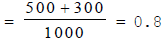(iv) P (less than two heads) = P (0 head or 1 head)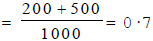(v) P (three heads)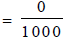(No chance favour the occurence of three heads) = 0

(vi) P (not more than two heads) =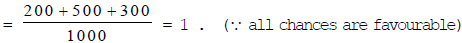Ex.5 A die is thrown 1000 times with the frequencies for the outcomes 1, 2, 3, 4, 5 and 6 as given
in the data below: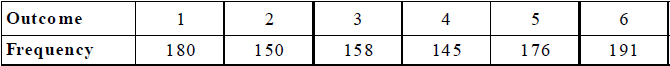Find the probability of getting each outcome. Also, show that the sum of the probabilities of all outcomes is 1.

Sol. We denote the event of getting 1 by E1 and similarly, E2, E3. E4. E5 and E6 for getting 2, 3, 4, 5 and 6
respectively. From the table, we have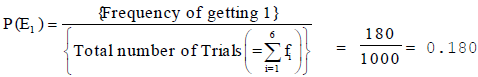Similarly,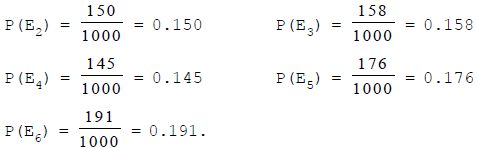Now,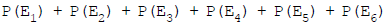= 0·180 + 0·150 + 0·158 + 0·145 + 0·176 + 0·191 = 1·000, i.e., 1.
Hence, the sum of the probabilities of all the outcomes is 1 .

Offer running on EduRev: Apply code STAYHOME200 to get INR 200 off on our premium plan EduRev Infinity!

,

,

,

,

,

,

,

,

,

,

,

,

,

,

,

,

,

,

,

,

,

,

,

,

,

,

,

;